# Microstructure evolution in deformed polycrystals predicted by a diffuse interface Cosserat approach

## Abstract

Formulating appropriate simulation models that capture the microstructure evolution at the mesoscale in metals undergoing thermomechanical treatments is a formidable task. In this work, an approach combining higher-order dislocation density based crystal plasticity with a phase-field model is used to predict microstructure evolution in deformed polycrystals. This approach allows to model the heterogeneous reorientation of the crystal lattice due to viscoplastic deformation and the reorientation due to migrating grain boundaries. The model is used to study the effect of strain localization in subgrain boundary formation and grain boundary migration due to stored dislocation densities. It is demonstrated that both these phenomena are inherently captured by the coupled approach.

## Introduction

A metallic polycrystal exposed to thermomechanical treatment can undergo significant microstructural evolution due to viscoplastic deformation and subsequent or concurrent recrystallization processes. Heterogeneous reorientation of the crystal lattice during plastic deformation may for instance lead to fragmentation of existing grains. In particular, material induced strain localization (slip bands) and curvature localization (kink bands) [1,2,3] may be associated with the formation of new (sub-)grain boundaries . The associated lattice curvature is accommodated by geometrically necessary dislocations (GNDs). At the same time, statistically stored dislocations (SSDs) accumulate in the microstructure due to random trapping . The associated stored energy is an important driving force for grain boundary migration [6,7,8,9], and in the recrystallization process it is observed that dislocation-free nuclei form which expand into and replace grains of high stored energy content. Detailed continuum descriptions of grain boundaries can also be based on decomposition into dislocation/disclination distributions  or micromechanical concepts allowing for shear-coupled boundary migration . These latter specific mechanisms are not addressed in the present work.

Viscoplastic deformation at the mesoscale (defined here as the grain scale of the polycrystal) including the heterogeneous reorientation discussed above can be successfully modeled by crystal plasticity approaches. In order to create extended frameworks which also take into account grain boundary migration after deformation, and in some cases nucleation as an intermediate step, several authors have coupled crystal plasticity models with methods such as cellular automata [12, 13], level-sets [14, 15] or phase-field methods [16,17,18,19,20] (these references are by no means a complete list but should rather be considered a sample of works for each mentioned approach). There are only a few works that consider a strong coupling, formulating and solving the complete set of equations as a monolithic system [21,22,23]. One of the major issues to resolve in a fully coupled framework is the fact that crystal orientation may change through deformation or through grain boundary migration. Often, the models that are combined rely on different descriptors of the microstructure and it is necessary to translate between those in intermediate processing steps.

Kobayashi, Warren and Carter (KWC) [24, 25] proposed a model that treats the crystal orientation as a phase-field with diffuse interfaces. Their formulation couples the evolution of the orientation phase-field to the evolution of a second, more classical phase-field variable. In this way, the model provides direct access to the change in crystal orientation that is due to atomic reshuffling processes in the wake of a migrating grain boundary. The energy density that KWC introduced only takes the norm of the orientation gradient (i.e., the lattice curvature) as a variable, and not the orientation itself, in order to respect the requirement of frame invariance. In particular, the inclusion of a linear term is necessary in order to produce a solution with regions of constant orientation (grains) separated by localized regions of non-zero curvature (grain boundaries). It is worth stressing that [26, 27] have amended this model with a contribution due to statistically stored dislocations, so as to address stored energy driven grain boundary migration that is the dominant growth phenomenon during recrystallization.

In this paper, a fully coupled, thermodynamically consistent model that combines a Cosserat single crystal plasticity model with the modified KWC orientation phase-field model of  is used to predict microstructure evolution in deformed polycrystals. The model was first developed for small deformations  and later for large deformations in . In this work, a linearized version of the large deformation model (c.f., ) is used. The Cosserat continuum is endowed with rotational degrees of freedom that can be associated with the lattice directors of the crystal. A strong coupling between the crystal plasticity model and the KWC orientation phase-field model is made possible via the Cosserat degrees of freedom. The lattice curvature parameter in the free energy density of the KWC model is replaced in the coupled model by the curvature or wryness tensor of the Cosserat continuum [29, 30].

Because the KWC model represents the lattice orientation as a continuous field rather than relying on a cellular description of the grains, it can accommodate the heterogeneous reorientation associated with plastic slip processes. Moreover, because the KWC model introduces a phase field variable, it is able to consider reorientation by a moving front. In the coupled model adopted in this work, the grain boundary migration is not accommodated by plastic slip processes, unlike the model in , where the KWC model is combined with strain gradient plasticity. Indeed, the driving forces for grain boundary migration are capillary forces or stored energy due to scalar dislocation densities which accumulate or recover following a modified Kocks–Mecking–Teodosiu evolution law [26,27,28].

This paper is organized as follows. The diffuse interface framework is summarized and the constitutive model is presented. A subsection is dedicated to the identification of model parameters. Then follows the numerical examples, with a subsection dedicated to the formation of (sub-)grain boundaries during deformation and a subsection dedicated to grain boundary migration. Some first results toward recrystallization are also presented. The paper is then concluded with a summary of the main results and some points on future work.

### Notation

Vectors will be denoted by underline as $$\underline{\varvec{a}}$$ and second order tensors by an underscore tilde symbol as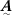, with the transpose. Third order tensors will in the same vein be denoted by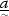and fourth order tensors by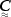. Double contraction is written as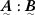and simple contraction as. The scalar product of two vectors can be written as $$\underline{\varvec{a}} \cdot \underline{\varvec{b}} = \underline{\varvec{a}}^T\,\underline{\varvec{b}}$$, whereas the dyadic product is written as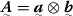. The gradient and divergence are written by products using the nabla symbol as $$\underline{\varvec{a}} \otimes \nabla$$ and $$\nabla \cdot \underline{\varvec{a}}$$ or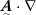, respectively, with differentiation of a tensor assumed to act on the second index. The curl operator is denoted.

The second order identity tensor will be denoted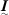. The transformations between skew-symmetric tensors and pseudo-vectors can be written by means of the Levi-Civita permutation tensor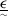as(1)

and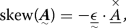(2)

respectively.

## The diffuse interface Cosserat framework

The small deformation theory presented in this section recalls the formulation proposed in , and represents the linearized version of the large deformation theory developed in .

### Governing equations

A Cosserat medium is endowed with three additional degrees of freedom at each material point, allowing for a microrotation which is, a priori, independent of the displacement degrees of freedom. The additional degrees of freedom necessary to describe the microrotation can be collected in the pseudo-vector $$\underline{\varvec{\varTheta }}$$. In the small deformation setting the Cosserat rotation tensor is then given by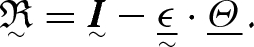(3)

The linearized, objective deformation measures are given by [29, 30]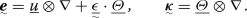(4)

where $$\underline{\varvec{u}}$$ is the displacement vector. The curvature or wryness tensor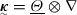can be directly related to the tensor of geometrically necessary dislocation densitiesthrough a famous result by Nye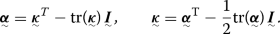(5)

The derivation of the above expression is detailed in .

In the framework of small deformations the Cosserat deformation tensor is decomposed into elastic and plastic parts as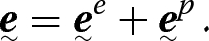(6)

So far, a similar decomposition of the curvature tensor has not been considered in the coupled theory although this would be possible to introduce as outlined in .

In order to allow for microstructure evolution due to grain boundary migration, [22, 28] coupled the Cosserat model to the orientation phase-field model proposed by [24, 25]. To this end, a phase-field $$\phi \in [0,1]$$ is introduced as an additional degree of freedom. The phase-field variable is interpreted in the coupled model as a coarse-grained measure of crystalline order. In the bulk of an undeformed grain the order parameter takes the value $$\phi = 1$$ whereas $$\phi < 1$$ in grain boundaries. In deformed grains the build-up of so-called statistically stored dislocations may also result in the phase-field variable taking values $$\phi < 1$$. This will be discussed in more detail when the constitutive model is presented. Note that the crystal orientation, which was included as a scalar phase-field in the model proposed by [24, 25], is associated with the Cosserat degrees of freedom in the model presented here.

The derivation of the governing balance equations and boundary conditions relies on the principle of virtual power and is detailed in [22, 28]. The phase-field contribution is accounted for in the principle of virtual power by the microstress formalism introduced by [33, 34]. There are therefore in total four work-conjugate pairs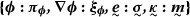and the principle of virtual power, after some manipulations, takes the form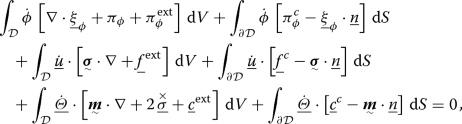(7)

over any region $$\mathcal {D}$$ of the body $$\Omega$$ with outward unit normal $$\underline{\varvec{n}}$$ on the boundary $$\partial \mathcal {D}$$. Superscripts $$\langle \bullet \rangle ^\mathrm{ext}$$ and $$\langle \bullet \rangle ^c$$ denote external body and contact forces and couples, respectively. It follows that the balance equations and corresponding boundary conditions for the coupled formulation are given by 

\begin{aligned}&\nabla \cdot \underline{\varvec{\xi }}_\phi + \pi _\phi + \pi _\phi ^{\mathrm{ext}} = 0 \quad \mathrm{in} \,\, \Omega \,, \end{aligned}
(8)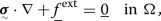(9)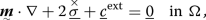(10)
\begin{aligned}&\underline{\varvec{\xi }}_\phi \cdot \underline{\varvec{n}} = \pi _\phi ^{c} \quad \mathrm{on} \,\, \partial \Omega \,, \end{aligned}
(11)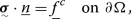(12)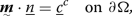(13)

where $$\underline{\varvec{n}}$$ is the outward normal to the boundary $$\partial \Omega$$ of the body $$\Omega$$. The first line (8) gives the balance law for the microstresses related to the phase field $$\phi$$ and its gradient $$\nabla \phi$$. It should be noted that the stressassociated with the Cosserat deformation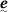is not symmetric, and must therefore not be interpreted as the usual Cauchy stress. Its skew-symmetric contribution appears also in the balance equation for the couple-stress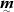, which in turn is associated with the wryness tensor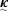. In this paper, the body forces $$\pi _\phi ^{\mathrm{ext}}, \underline{\varvec{f}}^{\mathrm{ext}}$$ and $$\underline{\varvec{c}}^{\mathrm{ext}}$$ are assumed to vanish.

Applying the standard thermodynamical principles on a free energy density of the format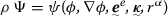, where $$r^\alpha$$ are internal variables related to the inelastic behavior, results in the following expression of the Clausius–Duhem inequality :(14)

assuming isothermal conditions. The constitutive relations are deduced from the energetic contribution to inequality (14):

\begin{aligned} \pi _\phi ^{eq}&= - \frac{\partial \psi }{\partial \phi } \,, \end{aligned}
(15)
\begin{aligned} \underline{\varvec{\xi }}_\phi&= \frac{\partial \psi }{\partial \nabla \phi } \,, \end{aligned}
(16)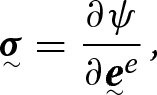(17)(18)

Furthermore, a thermodynamic force associated with the internal variables $$r^\alpha$$ can be introduced as

\begin{aligned} R^\alpha = \frac{\partial \psi }{\partial r^\alpha } \,. \end{aligned}
(19)

In order to recover the phase-field dynamics for the variable $$\phi$$, it is assumed in line with [34, 35] that the stress $$\pi _\phi$$ contains stored and dissipative contributions so that

\begin{aligned} \pi _\phi = \pi _\phi ^{eq} + \pi _\phi ^{neq} \,, \end{aligned}
(20)

with only the stored part given by (15).

Evolution equations governing the dissipative processes are assumed to be derivable from a potential (with the appropriate convexity properties to ensure non-negative dissipation)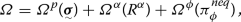(21)

so that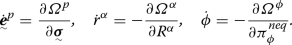(22)

### Link between the lattice rotation and the Cosserat microrotation

The rate of the Cosserat deformation can be written as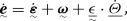(23)

where the symmetric contribution is the rate of the usual small strain tensor, and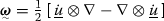is the spin tensor. The skew-symmetric part of the above expression can be collected in the pseudo-vector

\begin{aligned} \dot{\overset{\times }{\underline{\varvec{e}}}} = \overset{\times }{\underline{\varvec{\omega }}}- \dot{\underline{\varvec{\varTheta }}} \,. \end{aligned}
(24)

Equation (24) gives the relation between the relative rotation of the material and the Cosserat microrotation of a triad of microstructural directors attached to the material point.

By defining the elastic and plastic spin pseudo-vectors $$\overset{\times }{\underline{\varvec{\omega }}}\,^p := \dot{\overset{\times }{\underline{\varvec{e}}}}\,^p$$ and $$\overset{\times }{\underline{\varvec{\omega }}}\,^e := \overset{\times }{\underline{\varvec{\omega }}}-\overset{\times }{\underline{\varvec{\omega }}}\,^p$$, respectively, the rate of the skew-symmetric deformation (24) can now be expressed as

\begin{aligned} \overset{\times }{\underline{\varvec{\omega }}}\,^e - \dot{\underline{\varvec{\varTheta }}} = \dot{\overset{\times }{\underline{\varvec{e}}}}\,^e \,. \end{aligned}
(25)

The above equation provides the necessary link between the rotation of the crystal lattice vectors and the Cosserat directors that is an essential part of the modeling framework. They can be made to remain parallel by enforcing the internal constraint

\begin{aligned} \overset{\times }{\underline{\varvec{e}}}\,^e \equiv 0 \,. \end{aligned}
(26)

The Cosserat crystal plasticity framework thereby allows for a direct access to the heterogeneous evolution of the crystal orientation in the polycrystal during deformation.

## Constitutive model and identification of parameters

In this section the specific constitutive choices are presented and the identification of model parameters is described. Pure copper is used as a model material. The resulting model is a non-local Cosserat crystal plasticity model with diffuse and mobile grain boundaries. Grain boundary migration is either driven by the interface curvature or by the energy stored during plastic deformation.

### Constitutive model

The free energy density for the coupled problem is chosen as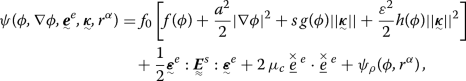(27)

with

\begin{aligned} \psi _\rho (\phi ,r^\alpha ) = \phi \sum _{\alpha =1}^N \frac{1}{2}\,\mu \,r^{\alpha 2} \,, \end{aligned}
(28)

where N is the number of crystal slip systems and $$r^\alpha$$ are internal variables. This contribution is taken to represent stored energy due to random trapping of dislocations during plastic deformation by associating the internal variables with the statistically stored dislocation densities, here denoted $$\rho ^\alpha$$, according to

\begin{aligned} r^\alpha = b \sqrt{\sum _{\beta = 1}^N h^{\alpha \beta } \rho ^\beta } \,. \end{aligned}
(29)

Inserted into Eq. (28), this then gives the following expression for the energy contribution related to the scalar dislocation densities $$\rho ^\alpha$$:

\begin{aligned} \psi _\rho (\phi ,r^\alpha ) = \frac{1}{2}\,\mu \,b^2\,\phi \sum _{\alpha =1}^N \sum _{\beta =1}^N h^{\alpha \beta } \rho ^\beta \,. \end{aligned}
(30)

This stored energy is an important driving force for grain boundary migration [7, 8]. The coupling with the phase-field variable by means of the linear term in $$\phi$$ ensures that local gradients in the stored energy result in grain boundary migration [26, 27]. In the grain boundary regions, $$\phi$$ tends toward zero. This means that energy is stored mainly in the bulk, and not inside the grain boundaries where the concept of statistically stored dislocations loses its significance.

Whereas the contribution due to the phase-field $$\phi$$ in the energy density (27) contains standard bulk and interphase terms, the contribution due to the curvature, which can be considered as a generalization to three dimensions of the energy contribution proposed by [24, 25] for the orientation phase-field, must contain the rank one term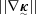. It is this term that ensures a solution with localized grain boundaries. At zero lattice curvature this term renders the energy density non differentiable and in the numerical treatment a regularization is therefore applied [28, 36]. In the context of non-local crystal plasticity, several authors have considered energy densities that contain linear contributions due to the GND density tensor [37,38,39], interpreting the linear term to represent the self energy of the GNDs whereas the quadratic term represents the energy due to their interactions. It may be noted that  found solutions with substructure partitioning, reminiscent of subgrains, even without a rank one term for a large deformation Cosserat crystal plasticity model. While those results are not directly applicable to the coupled model, it may nevertheless be warranted to reconsider the optimal format of the energy density in the future.

The functions $$g(\phi )$$ and $$h(\phi )$$ are required to be non-negative. A simple quadratic function is chosen for the latter such that $$h(\phi )=\phi ^2$$ whereas, following , a logarithmic function is chosen for $$g(\phi )$$ such that

\begin{aligned} g(\phi ) = -2\,\left[ \,\log (1-\phi ) + \phi \,\right] \,. \end{aligned}
(31)

This choice allows to fit a Read-Shockley type behavior for low angle grain boundaries. Due to the singularity for $$\phi =1$$, a small positive constant is added in the logarithm in the numerical treatment.

In the second line in (27), the symmetric elastic deformation is included via a standard quadratic form, whereis the usual elasticity tensor. The skew-symmetric elastic deformation is penalized by choosing the Cosserat parameter $$\mu _c$$ sufficiently large and thereby approaching the constraint (26) that the Cosserat microrotation should follow the lattice reorientation [30, 40,41,42,43]. This constraint could alternatively be directly imposed by using Lagrange multipliers.

The stress, couple stress and microstresses take the following forms: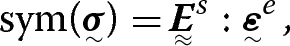(32)
\begin{aligned} \overset{\times }{\underline{\varvec{\sigma }}}= \,&2\,\mu _c \overset{\times }{\underline{\varvec{e}}}\,\!^e \end{aligned}
(33)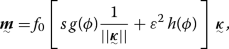(34)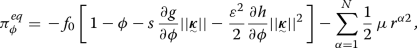(35)
\begin{aligned} \underline{\varvec{\xi }}_\phi = \,&f_0\, a^2 \nabla \phi \,. \end{aligned}
(36)

From (33) together with (24), it is apparent that a migrating grain boundary, which locally reorients the lattice as a result of atomic reshuffling processes, would give rise to a skew-symmetric stress. In order to relax this stress and allow the grain boundaries to migrate (without storing elastic energy), the plastic dissipation potential $$\varOmega ^p$$ is assumed to consist of two terms:(37)

where $$\langle \bullet \rangle = {\mathrm{Max}}(\bullet , 0)$$ and $$\overset{\times }{\underline{\varvec{\sigma }}}$$ contains the skew-symmetric contributions to the stress. The choice (28) results in a Taylor type hardening law for the associated force $$R^\alpha$$

\begin{aligned} R^\alpha = \frac{\partial \psi }{\partial r^\alpha } = \phi \, \,\mu \,r^{\alpha } = \phi \, \,\mu \,b \sqrt{\sum _{\beta = 1}^N h^{\alpha \beta } \rho ^\beta }\,, \end{aligned}
(38)

which is interpreted as the critical resolved shear stress on slip system $$\alpha$$. Equation (37) has been constructed so that the value of the phase-field $$\phi$$ will not influence the plastic slip flow evolution.

The first term of (37) leads to a classic crystal flow rule. The resolved shear stress $$\tau ^\alpha$$ on slip system $$\alpha$$ can be calculated as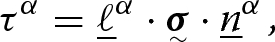(39)

where $$\underline{\varvec{\ell }}^\alpha$$ and $$\underline{\varvec{n}}^{\alpha }$$ are respectively the slip direction and normal to the slip plane. Note that the additional contribution to the resolved shear stress due to the non-symmetry of the stress tensor can be interpreted as a size-dependent kinematic hardening [4, 44,45,46]. The evolution of plastic deformation follows from (22) and (37)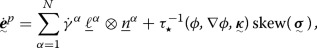(40)

with the slip rate given by

\begin{aligned} \dot{\gamma }^\alpha = \left\langle \,\frac{|\tau ^\alpha |-R^\alpha /\phi }{K_v} \, \right\rangle ^n \mathrm{sign} \, \tau ^\alpha \,. \end{aligned}
(41)

The second term in (40) allows the relaxation of the skew-symmetric stress by acting on the plastic spin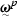. The viscosity type term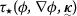is constructed so that it is small in the interface region but large in the bulk of the grains, thereby restricting the relaxation to the grain boundaries.

There are therefore two contributions to the plastic spin(42)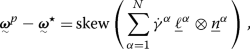(43)

due to the relaxation of the skew-symmetric stress at moving interfaces and to plastic slip processes, respectively. The skew-symmetric plastic deformation, likewise, can be considered to consist of two separate contributions

\begin{aligned} \overset{\times }{\underline{\varvec{e}}}\,\!^p = \overset{\times }{\underline{\varvec{e}}}\,\!^{slip} + \overset{\times }{\underline{\varvec{e}}}\,\!^\star \,, \end{aligned}
(44)

with

\begin{aligned} \dot{\overset{\times }{\underline{\varvec{e}}}}\,\!^{slip} = \overset{\times }{\underline{\varvec{\omega }}}\,\!^p - \overset{\times }{\underline{\varvec{\omega }}}\,\!^\star \,, \qquad \dot{\overset{\times }{\underline{\varvec{e}}}}\,\!^\star = \overset{\times }{\underline{\varvec{\omega }}}\,\!^\star \,. \end{aligned}
(45)

The elastic skew-symmetric deformation is then given by

\begin{aligned} \overset{\times }{\underline{\varvec{e}}}\,\!^e = \overset{\times }{\underline{\varvec{\vartheta }}}- \overset{\times }{\underline{\varvec{e}}}\,\!^{slip} - \overset{\times }{\underline{\varvec{e}}}\,\!^\star - \underline{\varvec{\varTheta }}\,, \end{aligned}
(46)

where $$\overset{\times }{\underline{\varvec{\vartheta }}}$$ is the pseudo-vector of the skew-symmetric tensor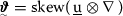denoting the material rotation.

In the present model, the Cosserat microrotation is associated with the lattice (crystal) orientation as measured with respect to some fixed frame. It is therefore in general non-zero in the reference configuration. It follows [21, 28] that the initial plastic deformation must be non-zero for the reference configuration to remain stress-free. Specifically, this is obtained by adopting the initial condition

\begin{aligned} \overset{\times }{\underline{\varvec{e}}}\,\!^p(t=0) = \overset{\times }{\underline{\varvec{e}}}\,\!^\star (t=0) = - \underline{\varvec{\varTheta }}(t=0) \,. \end{aligned}
(47)

The part of the inelastic deformation that is not due to slip processes during deformation can therefore be seen as necessary to accommodate an initial orientation distribution created from a previous, homogeneous state. It can be interpreted therefore, somewhat simplified, as representing a reference orientation state of the grain, and its associated evolution law ensures that it is inherited to a region swept by a migrating grain boundary. The evolution of the plastic deformation due to migrating grain boundaries according to Eq. (45) was reported in .

The standard evolution equation is obtained for the phase-field $$\phi$$ by choosing a quadratic dissipation potential [26, 33]

\begin{aligned} \varOmega ^\phi = \frac{1}{2} \tau _\phi ^{-1} \,\big (\,\pi _\phi ^{neq}\,\big )\,\!^2 \,, \end{aligned}
(48)

where $$\tau _\phi$$ is a viscosity type parameter, so that

\begin{aligned} \tau _\phi \, \dot{\phi }= - \pi _\phi ^{neq} \,. \end{aligned}
(49)

Instead of formulating a dissipation potential for the internal variables, which are associated with the scalar dislocation density in Eq. (29), modified Kocks–Mecking–Teodosiu evolution laws are used, given by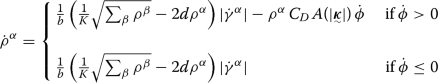(50)

The additional recovery term, with parameter $$C_D$$, was originally introduced by [26, 27]. It allows for static recovery behind the front of a migrating grain boundary, with the amount of recovery governed by the parameter $$C_D$$. The static recovery is localized to the interface region by the function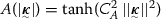. The competing nucleation and annihilation mechanisms in Eq. (50) determine whether energy is stored or dissipated. In particular, the additional recovery term allows for stored energy to be released through grain boundary migration.

### Identification of model parameters

The model parameters used in the simulations are given in Table 1. While typical values for most of the elasto-viscoplastic material parameters of pure copper at room temperature can be found in the literature (in this paper values adapted from [47, 48] are used), Table 1 also gives values for the recovery parameter $$C_D$$ in (50) and the Cosserat coupling modulus. The former is chosen so that full static recovery takes place in the wake of migrating grain boundaries. For the moment, only self interaction between static dislocations is considered. In Eq. (29), $$h_{\alpha \beta }=0.3$$ if $$\alpha =\beta$$ and otherwise $$h_{\alpha \beta }=0$$ if $$\alpha \ne \beta$$. The Cosserat modulus is chosen sufficiently large to act as a numerical penalty parameter.

The parameters $$f_0$$, a, and s, related to the phase-field inspired energy contribution in (27), are calibrated to fit values obtained from atomistic calculations for $$<100>$$ tilt grain boundaries. Following , the general calibration procedure relies on the matched asymptotic analysis of the original KWC model as proposed in . At the lowest order corresponding to equilibrium, the coupled model becomes equivalent to the KWC model. Hence, using the results from , it is possible to calculate the grain boundary energy as a function of the misorientation angle. Some grain boundary energies—calculated by atomistic simulations [50,51,52] for copper tilt grain boundaries—are collected in Fig. 1 (top). The grain boundary energy exhibits cusps, i.e., favored misorientations which result in local energy minima. The current model does not take into consideration such local minima so the calibration was only performed on the first part of the curve up to a misorientation angle of 30$$^\circ$$. The result is shown with stars in Fig. 1 (bottom) and the calibrated parameters are given in Table 1. The parameter $$\varepsilon$$ controls (together with a) the width of the grain boundary. It is chosen to be $$\varepsilon = 2$$$$\upmu \mathrm {m}$$, which results in a diffuse interface width of around 500 nm. The interface width puts a restriction on the element size in the finite element discretization. At least four to five nodes, but ideally more, are required to resolve the curvature.

Whereas the coupled Cosserat model and the model proposed in  (which is close to the original KWC model) become similar at equilibrium, they differ in dynamic situations. The two formulations have been compared in [21, 28]. The model in  relies on a relaxation dynamics for the orientation, whereas in the coupled model the orientation is at each instant governed by the constraint (46) together with the balance equation (34). Because of this difference, it is not possible to use the asymptotic analysis of the KWC model to calibrate the mobility parameters of the coupled model, because only the expansion to lowest order is valid in this case.

The choice of the parameterin Eq. (42) was studied in  where it was shown that it must be bounded by the choice of $$\tau _\phi$$ in order not to restrict the grain boundary mobility. It will be assumed to be of the general form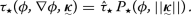(51)

The function, which is meant to localize the stress relaxation to the interface region, will be discussed in the next section. In concordance with , the parameter $$\hat{\tau }_\star$$ is chosen to be $$\tau _\phi /10$$. Both values are given (scaled by $$f_0$$ for clarity) in Table 1. The choice of the parameters for the dynamic behavior is revisited in the next section when the numerical simulations are discussed. A formal procedure for identifying the dynamic coefficients of the model remains to be established, which is why in this work the mobility parameter $$\tau _\phi$$ is adjusted by running numerical simulations on simplified test cases.

## Microstructure evolution in deformed polycrystals

In this section, the microstructure evolution predicted by the proposed model in deformed polycrystals is investigated by numerical simulations. In particular, the effect of localized reorientation of the lattice and the grain boundary migration due to statistically stored dislocation densities are studied. The numerical simulations are performed using the finite element code Z-set . The discretization of the weak form of the balance equations results in a monolithic, coupled system of equations which is solved using implicit integration in an iterative Newton–Raphson scheme. The constitutive equations are integrated using a fourth order Runge-Kutta method with automatic time-stepping. For details on the numerical implementation, see [22, 28] and also [26, 41] and for the Cosserat crystal plasticity and the phase-field model, respectively. Since only planar problems are considered, $$\underline{\varvec{\varTheta }}= [\begin{array}{ccc} 0&0&\theta \end{array}]^T$$, with $$x_3$$ considered to be the out-of-plane axis and $$\theta _3=\theta$$ the only non-zero orientation degree of freedom. The planar problem therefore has only four degrees of freedom: two components of displacement, the microrotation $$\theta$$, associated with the crystal orientation, and the phase-field $$\phi$$.

Periodic geometries and boundary conditions are considered. Simple shear loading is then applied by imposing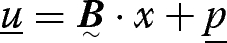(52)

with the tensor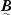given by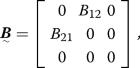(53)

with only either $$B_{12}$$ or $$B_{21}$$ non-zero. The vector $$\underline{\varvec{p}}$$ is a periodic fluctuation that takes the same value in opposite points of the boundary.

### Grain boundary mobility

As discussed in the previous section, the grain boundary mobility cannot be calibrated based on results for the orientation phase-field model, as the evolution equations for the two models are different. While a formal calibration procedure remains to be established, the grain boundary mobility can be estimated and adjusted by running a simple test case. This test case is a periodic bigrain of the type considered in , with a flat grain boundary and 15$$^\circ$$ misorientation between the two grains. One grain is assigned a (constant) scalar dislocation density and the other grain is assumed to be dislocation-free. The resulting stored energy gradient acts as a driving force for grain boundary migration. With a planar grain boundary and a constant stored energy gradient, the grain boundary mobility can be calculated as $$\mathcal {M} = v/\psi _\rho$$, with v being the grain boundary velocity and $$\psi _\rho$$ the pressure acting on the grain boundary due to the stored energy.

In the viscosity type parameterin Eq. (51), the localizing function is chosen to be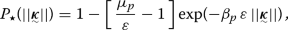(54)

following . In , the same localizing function was used for the orientation phase-field, whereas a $$\tanh$$ function was used for the $$\tau _\star$$ coefficient. The constant $$\mu _p$$ determines the value of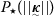in the bulk of the grains and should be chosen to be large so that $$1/\tau _\star \rightarrow 0$$ there. The constant $$\beta _p$$ sets the width of the region around the grain boundary whereand should be chosen $$\beta _p \le 10^3$$ in order to achieve localization. In the simulations, $$\mu _p=10^9$$ $$\upmu \mathrm {u}$$   and $$\beta _p=10^2$$ are used.

With the driving pressure due to the stored dislocation density given by $$\psi _\rho =0.23$$ MPa and using the material parameters given in Table 1, the resulting simulated grain boundary velocity is $$v = 0.8$$ nm/s. The resulting mobility is $$\mathcal {M} = 3.5$$ nm/(MPa s). While there is not a plethora of available experimental data on the grain boundary mobility,  measured an average grain boundary mobility of 0.61 nm/(MPa s) at 121 $$^\circ$$C for recrystallization of cold deformed copper. Theoretical average grain boundary mobilities were also calculated in . Compared to that data, $$\mathcal {M} = 3.5$$ nm/(MPa s) corresponds to recrystallization at a temperature of approximately 150–160 $$^\circ$$C. In the present work, thermal effects are not accounted for in the simulations, and the indicated temperature range should not be considered predictive of the true final temperature of the system.

In order to compare the behavior of the coupled model and the orientation phase-field model, the latter was also used to run the same example of a periodic bigrain with a stored energy gradient. The model parameters, where applicable, were the same as for the coupled model, and in the evolution equation for the orientation phase-field (c.f. [24, 26, 28]), the mobility parameter was chosen identical to. The mobility predicted by that simulation was $$\mathcal {M} = 0.87$$ nm/(MPa s), which is lower than for the coupled model. This is as expected and further indicates that it is necessary to establish a separate calibration procedure for the mobility of the coupled model.

### Initial conditions for polycrystal simulations

In general, initial microstructures for polycrystalline aggregates are obtained from measurements of real samples, or generated by tessellation using dedicated software such as Neper . The tessellations can then be translated into a finite element mesh with discrete regions of different orientation, i.e., grains. In this work, two periodic, planar microstructures generated with Neper are used, one with six grains and one with 32 grains. The smaller geometry is $$20 \times 20$$ $$\upmu \mathrm {m}$$  in size, discretized with 8464 ($$92 \times 92$$) elements with quadratic interpolation functions and reduced integration. The larger geometry is $$50 \times 50$$ $$\upmu \mathrm {m}$$, and it is discretized with 47089 ($$217 \times 217$$) elements. The element size is therefore nearly the same in both examples, 0.21 $$\upmu \mathrm {m}$$  in the six grains geometry compared to 0.23 $$\upmu \mathrm {m}$$  in the one with 32 grains. The orientations are distributed in the range $$0^\circ$$ to $$35^\circ$$, which corresponds to the range of misorientations in the grain boundary energy for which the model is calibrated.

The grain morphologies and orientation distributions obtained using Neper are not suitable for use as initial conditions in the coupled model as they are far from the equilibrium solution, with jagged edges tied to the finite element grid, and so will result in convergence problems (Fig. 2, left). In order to generate suitable initial conditions for the non-zero component $$\theta$$ of $$\overset{\times }{\underline{\varvec{\varTheta }}}$$ as well as $$\phi$$, simulations using the KWC orientation phase-field model are performed. This will also generate initial conditions for the non-zero component $$\overset{\times }{e}\,\!^p$$ of $$\overset{\times }{\underline{\varvec{e}}}\,\!^p$$ (c.f., Eq. (47)). The simulations take the grain morphologies generated by Neper as initial conditions, together with a uniform $$\phi =1$$. The simulations are run until a solution with localized, smooth grain boundaries is found. Figure 2 shows the microstructure generated by Neper for the geometry with six grains and the corresponding output for the orientation field from the phase-field simulation. This field will serve as initial conditions on $$\theta$$ and -$$\overset{\times }{e}\,\!^p$$ in the coupled simulations, as shown in Fig. 3 (top left). The phase-field simulations also find the corresponding solution for $$\phi$$, shown in Fig. 3 (bottom left). Initial conditions for the geometry with 32 grains are generated by the same procedure. In all simulations, the dislocation densities have a uniform initial value of $$\rho ^\alpha =2\cdot 10^{11}$$ m$$^{-2}$$.

### Kink bands and subgrain formation

Up to four possible slip systems are considered for the planar problem with the slip directions given by

\begin{aligned} \begin{aligned}&\underline{\varvec{l}}^1 = \left( \,1,0\,\right) \,,&\quad&\underline{\varvec{l}}^2 = \left( \,0,1\,\right) \\&\underline{\varvec{l}}^3 = \frac{1}{\sqrt{2}} \left( \,1,1\,\right) \,,&\quad&\underline{\varvec{l}}^4 = \frac{1}{\sqrt{2}} \left( \,-1,1\,\right) \,. \\ \end{aligned} \end{aligned}
(55)

The slip plane normal in each case is perpendicular to the slip direction. By choosing which slip systems are allowed to be active in a simulation, localization phenomena such as kink or slip bands can be triggered. In particular, the formation of kink bands, which are bands of localized plastic deformation that are perpendicular to the slip direction, can result in large localized reorientation of the lattice (see the recent contribution  for a detailed description of kink and slip bands in crystals and how they arise in crystal plasticity simulations). In the simulations, a periodic shear deformation is applied with $$B_{21} = 0.05$$ in Eq. (52). Kink bands are triggered when only one slip system can be activated.

Figure 3 shows the orientation field $$\theta$$ (top), the phase-field $$\phi$$ (middle) and the curvature norm $$|\nabla \theta |$$ (bottom) before (left) and after (right) deformation of the six grains microstructure. The deformation is not shown, i.e., the fields are projected onto the undeformed mesh (the total shear deformation is five percent). Only slip system $$\alpha =2$$ was allowed to activate in the simulation. The formation of kink bands is most easily seen in the bottom right figure, which shows the norm of the curvature $$|\nabla \theta |$$ in the deformed structure. These bands are also visible in the phase-field $$\phi$$. Note that the grains are oriented between $$0^\circ \mathrm {and}\, 35^\circ$$ from the $$x_1$$-direction, so the localized bands are perpendicular to the slip direction since it is the (0, 1) slip direction that is active. In the top left image, the slip direction of each grain is indicated with black lines. The orientation phase-field method always localizes grain boundaries where there is a sufficient orientation gradient , and clearly this property is inherited to the coupled model, as may be expected. In particular, a kink band has formed across the bottom part of the left central (green) grain and the right central (blue) grains, resulting in what appears to be two, maybe three, new grain boundaries delimiting new subgrains. The locations of the new grain boundaries are near the spots marked 1 and 2, respectively, in the top right image. Here, the term subgrain is used to denote regions that result from partitioning of the original grain structure due to the deformation. The model automatically handles localization and, while it is sensitive to the magnitude of the orientation gradient, does not differentiate between different types of grain boundaries.

Figure 4 shows the results from calculations when only slip system $$\alpha =1$$ was allowed to activate. Kink band formation is again observed but the localization is less pronounced than in the previous example. Slip band formation is not observed. The figure shows the orientation field $$\theta$$ (top), the phase-field $$\phi$$ (middle) and the curvature norm $$|\nabla \theta |$$ (bottom) before (left) and after (right) deformation.

Figure 5 shows the same results as Fig. 3, but for the larger geometry with 32 grains. Again, the formation of kink bands is most notable in the contour map showing the norm of the orientation gradient $$|\nabla \theta |$$ in the deformed microstructure (bottom right), but it is also visible in the phase-field $$\phi$$ (middle right). In particular, below the spot marked with 1 in the top right image, a little bit above the center of the geometry, there are two new subgrains that seem to have formed due to the reorientation at the edges of two existing grains. The simulation shows how a kink band associated with a strong lattice curvature transforms into a true grain boundary, which is associated with a significant decrease in $$\phi$$ due to the large accumulation of dislocation densities.

The interest of these examples is that by triggering kink deformation in the simulations, it is possible to study how the model behaves when very localized reorientation takes place. Clearly, the coupled framework allows for the formation of new subgrains by reorientation without the need for post-processing or reinterpretation of the results.

### Dislocation driven grain boundary migration

During the elasto-viscoplastic deformation, energy is stored in the bulk of the grains due to random trapping of dislocations. This is included in the model via the energy term (28) and the modified Kocks–Mecking–Teodosiu evolution law (50) for the evolution of the scalar dislocation densities $$\rho ^\alpha$$. Because the energy contribution (28) is coupled with $$\phi$$ in the energy density, a difference in stored energy between two grains contributes to grain boundary migration. This effect is illustrated by comparing simulations performed with only one active slip system (the same as in the previous example) or all four slip systems of (55). In the former case, the difference in stored energy is higher, and correspondingly there is a stronger contribution to the microstructure evolution due to the statistically stored dislocation densities.

Figure 6 shows the accumulated plastic strain (left) and static dislocation density (right) in the case of only one active slip system for the six grain geometry. The maximum scalar dislocation density is in reality $$2.4\cdot 10^{15}$$ m$$^{-2}$$, but in the figure the maximum display value has been limited to $$5\cdot 10^{14}$$ m$$^{-2}$$ in order to better highlight how the dislocation density is distributed between different grains. In Fig. 7, the scaled stored energy $$\psi _\rho /f_0$$ with one (left) or four (right) active slip systems is shown. When all four slip systems are active, energy is stored much more evenly in the grains. The maximum value is around 0.9 for one possible slip system, whereas it is only around 0.1 with four possible slip systems, so its contribution to the driving force for grain boundary migration in the latter case is considerably smaller. Note that in Fig. 7, the maximum display value in the left figure (one possible slip system) has been limited to 0.5 in order to better represent how the stored energy is distributed in the different grains.

The microstructure evolution is studied by applying a shear deformation and then holding it constant for 2 h under a moderately elevated temperature. The grain boundary mobility was chosen to correspond with a (constant) temperature of around 150 $$^\circ$$C. This value is not predictive of the true temperature of the system; it would be necessary to fully take into account thermal effects to achieve this. The final microstructure for the six grains microstructure is shown in Fig. 8 for one (left) or four (right) possible active slip systems. The images show, from top to bottom, the orientation field, the phase-field $$\phi$$ and the curvature $$|\nabla \theta |$$, respectively. The initial configuration is the same for both cases and the initial grain boundaries are outlined in black.

It is clear that the predicted grain structures are very different. With only one active slip system, it seems that the grain boundary migration is primarily driven by the difference in stored energy associated with statistically dislocation densities. With four possible slip systems on the other hand, it appears that the grain boundary migration is driven mostly by the grain boundary curvature. This is evident from the shrinking of the smallest grain, which has a slightly lower stored energy than its neighbor to the left and therefore should expand in that direction if this had been the main driving force. Instead, it shrinks to lower the energy in the triple junction. It is also clear from comparing the two examples that the stored energy due to the scalar dislocation densities is a stronger driving force than curvature for grain boundary migration in this case. This is in agreement with the typical driving pressures given in , with typical driving pressures due to stored dislocations generally several magnitudes larger than those due to the grain boundary energy.

To study the evolution of the statistically stored dislocations, the simulation with one possible active slip system is considered again. In Fig. 9, the dislocation density in the grains is compared at two time points: just after the deformation is applied (left) and after 2 h (right). The grain with the highest dislocation density post deformation has vanished completely after 2 h, and in the wake of the migrating grain boundaries, static recovery has left a region free of statistically stored dislocations.

The larger geometry with 32 grains is also studied. Figure 10 shows, from left to right, the orientation field, the phase-field $$\phi$$ and the norm of the curvature $$|\nabla \theta |$$ after 1 h of final deformation maintained constant. The initial conditions and the corresponding fields immediately after the deformation is applied are given in Fig. 5. The microstructure has evolved relatively little in the time interval considered. In part, this may be due to the distribution of the stored energy inside the grains, which may be less favorable to grain boundary migration than in the smaller sample. The maximum value of the stored energy is also lower than in the previous example, approximately $$1.9\cdot 10^{15}$$ m$$^{-2}$$ (in Fig. 11, the maximum display value for $$\rho$$ has been set to $$5\cdot 10^{14}$$ m$$^{-2}$$). In the smaller sample, most of the microstructure evolution takes place within the first hour, so the shorter time span considered in the larger example is not in itself sufficient to explain the difference.

### Observed phenomena for further investigation

For both considered geometries, there are some phenomena that appear in the simulations that may require further investigation. Figure 12 shows a zoom on the subgrain boundary that has formed in the lower left corner of the six grain geometry after deformation, c.f. Figs. 3 and 6. Only one slip system is allowed. From top to bottom the images show the orientation, the dislocation density $$\rho$$, the norm of the curvature $$|\nabla \theta |$$, the phase-field $$\phi$$, and the resolved shear stress $$\tau$$ (ranging from $$-\,160$$ to 230 MPa). The time evolution is shown from left to right: just after deformation and then after 5 min, 1 h, and 2 h of deformation.

Already immediately after the deformation is applied, it seems that the reoriented bands (blue above and yellow below) are not uniform and that there are small spots of darker color dispersed inside them. Already after 5 min, and even more so after 1 h, the reoriented bands have mostly vanished (due to local reorientation) except for these spots. Furthermore, in the same position, there appears in the scalar dislocation density small regions where static recovery has taken place but without apparent grain boundary migration (recovery in connection with grain boundary curvature on the other hand seems to have taken place to the right of the region of interest, where the grain to the right has grown into the grain to the left). There is some, but very little additional change between $$t=1$$ h and $$t=2$$ h, and there is no definite indication for the moment that these small regions will eventually expand.

The same behavior can be seen for the larger simulation geometry in Figs. 10 and 11. There are two grains a little bit above the center (below the spot marked with 1 in Fig. 5, top right) where there is significant local reorientation and a similar pattern of spots appear in the orientation field (Fig. 10 left) and corresponding regions of apparent static recovery in the dislocation density (Fig. 11 right). In order to study if this behavior is due to poor resolution of the localization taking place in the kink bands, a second simulation was performed for the six grain geometry with a finer mesh with 20,449 (143 by 143) elements (the coarser mesh has 8464 elements). The same type of behavior is again observed, as shown in Fig. 13. It is thus present in two different geometries, and for the same geometry with two different finite element sizes. Further investigation is necessary to determine the precise origin of the irregularities in the orientation distribution, and if it is possible that they could act as seeds for new grains and subsequent recrystallization. The evolution of the other fields, notably the scalar dislocation density, can be explained by the time evolution predicted by the model as a consequence of the heterogeneities in the microstructure. The phase field $$\phi$$ always follows the orientation field, as indicated in the previous examples, and this also activates the static recovery term in the modified Kocks–Mecking–Teodosiu equations (50).

The second phenomena that may warrant further investigation appears in the 32 grain geometry, where one grain boundary exhibits signs of bulging. The grain boundary can be located in Fig. 5 (top right) where it is near the spot marked with a 2. This is shown in Figs. 14 and 15. The grain has a boundary near the edge of the simulation box (in the periodic geometry), and so the change in the profile to the very right in the figures is likely due to ordinary grain boundary migration. However, a little to the left there is a small bulge that appears which can not be explained by the geometry. This bulge is apparent in both the orientation field in Fig. 14 and in the phase-field $$\phi$$ in Fig. 15 (top). In addition, it seems to be associated with static recovery of the dislocation density $$\rho$$, shown in Fig. 15 (bottom). Again there is not much evolution however after the bulge initially appears. It seems to stay at the same size between 1000 s and 1 h in Fig. 14. It is likely that it will be eaten up by the moving front to the right before it evolves much further.

## Summary and conclusions

Microstructure evolution in deformed polycrystals is studied in this work by finite element simulations, applying a constitutive model that combines Cosserat single crystal plasticity with a phase-field model. It is shown that the model is capable of taking into account significant microstructural changes. A major result is the importance of strain localization (slip bands) and curvature localization (kink bands) on subsequent grain boundary formation and grain boundary migration. Simulations with only one possible slip system are used to trigger the formation of kink bands and obtain curvature localization. There is some clear evidence in the results of new grain boundaries appearing—that is to say: it is demonstrated that the phase-field variable is sensitive to regions of high dislocation density and that the coupled simulation model has inherited the quality of the KWC orientation phase-field model to localize grain boundaries in regions of strong curvature.

Grain boundary migration is also present in the simulation results, both due to stored energy (SSD densities) and to curvature. The most important driving pressures for grain boundary migration are obtained when large stored energy gradients occur between individual grains.

The presented simulations are still at their early stage (2D, small strain and rotations) but they indicate that the model contains physical ingredients that are responsible for subgrain boundary formation, formation of possible recrystallization seeds, and evidence of bulging phenomenon. It will be necessary in a first step, which is a current focus, to extend the model to perform more realistic 3D simulations. The full 3D version of the model requires seven degrees of freedom at each material point, rather than four in the 2D case. The increase in numerical cost is therefore very significant. The main challenge, however, is the representation of the orientation field in 3D. In the plane case, the grain orientation is treated as a scalar field, with rotation only around one major axis. The fact that the orientation is restricted to the plane in the 2D version of the model severely limits the types of slip systems that can be studied, which will not be the case in a 3D formulation. However, if rotation around several axes is considered, the interpolation of the variation of orientation between two grains requires some consideration.

The large deformation formulation of the model has already been established  and in a second step, its numerical implementation will allow to study processes such as cold working and subsequent annealing but also to tackle the more complex problem of dynamic recrystallization with concurrent deformation, nucleation and grain growth. This would for example allow to investigate if the model can predict a real threshold in stored energy for strain induced grain boundary migration to take place. While the simulations performed in the present study showed some tendency toward grain boundary bulging, it is not clear whether a critical work-hardening can be an outcome of the model or if the model must be enhanced.

Not applicable.

## References

1. Asaro RJ, Rice JR. Strain localization in ductile single crystals. J Mech Phys Solids. 1977;25(5):309–38. https://doi.org/10.1016/0022-5096(77)90001-1.

2. Forest S. Modeling slip, kink and shear banding in classical and generalized single crystal plasticity. Acta Mater. 1998;46(9):3265–81. https://doi.org/10.1016/S1359-6454(98)00012-3.

3. Marano A, Gélébart L, Forest S. Intragranular localization induced by softening crystal plasticity: analysis of slip and kink bands localization modes from high resolution FFT-simulations results. Acta Mater. 2019;15:262–75. https://doi.org/10.1016/j.actamat.2019.06.010.

4. Sedláček R, Blum W, Kratochvil J, Forest S. Subgrain formation during deformation: physical origin and consequences. Metall Mater Trans. 2002;33A:319–27.

5. Ashby MF. The deformation of plastically non-homogeneous materials. Philos Mag. 1970;21:399–424.

6. Bever MB, Holt DL, Titchener AL. The stored energy of cold work. Prog MaterSci. 1973;17:5–177. https://doi.org/10.1016/0079-6425(73)90001-7.

7. Humphreys FJ, Hatherly M. Recrystallization and related annealing phenomena. Amsterdam: Elsevier; 2004.

8. Gottstein G, Shvindlerman LS. Grain boundary migration in metals. Berlin: CRC Press; 2010.

9. Renk O, Hohenwarter A, Wurster S, Pippan R. Direct evidence for grain boundary motion as the dominant restoration mechanism in the steady-state regime of extremely cold-rolled copper. Acta Mater. 2014;77:401–10. https://doi.org/10.1016/j.actamat.2014.06.010.

10. Taupin V, Capolungo L, Fressengeas C, Das A, Upadhyay M. Grain boundary modeling using an elasto-plastic theory of dislocation and disclination. J Mech Phys Solids. 2013;61:370–84.

11. Berbenni S, Paliwal B, Cherkaoui M. A micromechanics-based model for shear-coupled grain boundary migration in bicrystals. Int J Plast. 2013;44:68–94.

12. Raabe D, Becker RC. Coupling of a crystal plasticity finite-element model with a probabilistic cellular automaton for simulating primary static recrystallization in aluminium. Model Simul Mater Sci Eng. 2000;8(4):445.

13. Popova E, Staraselski Y, Brahme A, Mishra RK, Inal K. Coupled crystal plasticity—probabilistic cellular automata approach to model dynamic recrystallization in magnesium alloys. Int J Plast. 2015;66:85–102. https://doi.org/10.1016/j.ijplas.2014.04.008.

14. Hallberg H. A modified level set approach to 2D modeling of dynamic recrystallization. Modell Simul Mater Sci Eng. 2013;21(8):085012. https://doi.org/10.1088/0965-0393/21/8/085012.

15. Blesgen T. A variational model for dynamic recrystallization based on Cosserat plasticity. Compos Part B Eng. 2017;115:236–43.

16. Takaki T, Yamanaka A, Higa Y, Tomita Y. Phase-field model during static recrystallization based on crystal-plasticity theory. J Comput Aided Mater Des. 2007;14(1):75–84.

17. Takaki T, Hisakuni Y, Hirouchi T, Yamanaka A, Tomita Y. Multi-phase-field simulations for dynamic recrystallization. Comput Mater Sci. 2009;45(4):881–8.

18. Güven O, Henke T, Laschet G, Böttger B, Apel M, Bambach M, Hirt G. Modelling of static recrystallization kinetics by coupling crystal plasticity FEM and multiphase field calculations. Comput Methods Mater Sci. 2013;13(2):368–74.

19. Güven O, Bambach M, Hirt G. Coupling of crystal plasticity finite element and phase field methods for the prediction of SRX kinetics after hot working. Steel Res Int. 2014;85(6):999–1009.

20. Vondrous A, Bienger P, Schreijäg S, Selzer M, Schneider D, Nestler B, Helm D, Mönig R. Combined crystal plasticity and phase-field method for recrystallization in a process chain of sheet metal production. Comput Mech. 2015;55(2):439–52.

21. Ask A, Forest S, Appolaire B, Ammar K. Cosserat crystal plasticity with dislocation-driven grain boundary migration. J Micromech Mol Phys. 2018;03(03n04):1840009. https://doi.org/10.1142/S242491301840009X.

22. Ask A, Forest S, Appolaire B, Ammar K. A cosserat-phase field theory of crystal plasticity and grain boundary migration at finite deformation. Contin Mech Thermodyn. 2018;31(4):1109–41. https://doi.org/10.1007/s00161-018-0727-6.

23. Admal NC, Po G, Marian J. A unified framework for polycrystal plasticity with grain boundary evolution. Int J Plast. 2018;. https://doi.org/10.1016/j.ijplas.2018.01.014.

24. Kobayashi R, Warren JA, Carter WC. A continuum model of grain boundaries. Phys D. 2000;140(1–2):141–50.

25. Warren JA, Kobayashi R, Lobkovsky AE, Carter WC. Extending phase field models of solidification to polycrystalline materials. Acta Mater. 2003;51(20):6035–58.

26. Abrivard G, Busso EP, Forest S, Appolaire B. Phase field modelling of grain boundary motion driven by curvature and stored energy gradients. Part I: theory and numerical implementation. Philos Mag. 2012;92:3618–42.

27. Abrivard G, Busso EP, Forest S, Appolaire B. Phase field modelling of grain boundary motion driven by curvature and stored energy gradients. Part II: application to recrystallisation. Philos Mag. 2012;92:3643–64.

28. Ask A, Forest S, Appolaire B, Ammar K, Salman OU. A cosserat crystal plasticity and phase field theory for grain boundary migration. J Mech Phys Solids. 2018;115:167–94. https://doi.org/10.1016/j.jmps.2018.03.006.

29. Eringen AC, Kafadar CB. Part I. Polar field theories. In: Eringen AC, editor. Continuum physics. New York: Academic Press; 1976. p. 1–73.

30. Forest S, Cailletaud G, Sievert R. A Cosserat theory for elastoviscoplastic single crystals at finite deformation. Arch Mech. 1997;49(4):705–36.

31. Nye JF. Some geometrical relations in dislocated crystals. Acta Metall. 1953;1:153–62.

32. Forest S, Sievert R. Elastoviscoplastic constitutive frameworks for generalized continua. Acta Mech. 2003;160:71–111.

33. Gurtin ME. Generalized Ginzburg–Landau and Cahn–Hilliard equations based on a microforce balance. Phys D Nonlinear Phenom. 1996;92(3):178–92.

34. Gurtin ME, Lusk MT. Sharp-interface and phase-field theories of recrystallization in the plane. Phys D Nonlinear Phenom. 1999;130(1–2):133–54.

35. Gurtin ME. A gradient theory of single-crystal viscoplasticity that accounts for geometrically necessary dislocations. J Mech Phys Solids. 2002;50(1):5–32.

36. Kobayashi R, Giga Y. Equations with singular diffusivity. J Stat Phys. 1999;95(5–6):1189–220.

37. Ohno N, Okumura D. Higher-order stress and grain size effects due to self-energy of geometrically necessary dislocations. J Mech Phys Solids. 2007;55:1879–98.

38. Mesarovic SD, Forest S, Jaric JP. Size-dependent energy in crystal plasticity and continuum dislocation models. Proc R Soc A Math Phys Eng Sci. 2015;471(2175):20140868.

39. Wulfinghoff S, Forest S, Böhlke T. Strain gradient plasticity modeling of the cyclic behavior of laminate microstructures. J Mech Phys Solids. 2015;79:1–20. https://doi.org/10.1016/j.jmps.2015.02.008.

40. Blesgen T. Deformation patterning in three-dimensional large-strain Cosserat plasticity. Mech Res Commun. 2014;62:37–43.

41. Forest S, Barbe F, Cailletaud G. Cosserat modelling of size effects in the mechanical behaviour of polycrystals and multiphase materials. Int J Solids Struct. 2000;37:7105–26.

42. Mayeur JR, McDowell DL, Bammann DJ. Dislocation-based micropolar single crystal plasticity: comparison of multi- and single criterion theories. J Mech Phys Solids. 2011;59:398–422.

43. Mayeur JR, McDowell DL. A comparison of gurtin type and micropolar theories of generalized single crystal plasticity. Int J Plast. 2014;57:29–51. https://doi.org/10.1016/j.ijplas.2014.01.010.

44. Sedláček R, Forest S. Non-local plasticity at microscale: a dislocation-based model and a Cosserat model. Phys Status Solidi (B). 2000;221:583–96.

45. Forest S, Sedláček R. Plastic slip distribution in two-phase laminate microstructures: dislocation-based vs. generalized-continuum approaches. Philos Mag A. 2003;83:245–76.

46. Forest S. Some links between Cosserat, strain gradient crystal plasticity and the statistical theory of dislocations. Philos Mag. 2008;88:3549–63.

47. Gérard C, Bacroix B, Bornert M, Cailletaud G, Crépin J, Leclercq S. Hardening description for FCC materials under complex loading paths. Comput Mater Sci. 2009;45(3):751–5. https://doi.org/10.1016/j.commatsci.2008.08.027.

48. Cheong K-S, Busso EP. Discrete dislocation density modelling of single phase FCC polycrystal aggregates. Acta Mater. 2004;52(19):5665–75. https://doi.org/10.1016/j.actamat.2004.08.044.

49. Lobkovsky AE, Warren JA. Sharp interface limit of a phase-field model of crystal grains. Phys Rev E. 2001;63(5):051605.

50. Wolf D. Structure-energy correlation for grain boundaries in FCC metals—III. Symmetrical tilt boundaries. Acta Metall Mater. 1990;38(5):781–90. https://doi.org/10.1016/0956-7151(90)90030-K.

51. Missol litold. Orientation dependence of grain-boundary energy in metals in the view of a pseudoheterophase dislocation core model. Acta Metall. 1976;24(1):73–9. https://doi.org/10.1016/0001-6160(76)90149-8.

52. Tschopp MA, Coleman SP, McDowell DL. Symmetric and asymmetric tilt grain boundary structure and energy in Cu and Al (and transferability to other FCC metals). Integr Mater Manuf Innov. 2015;4(1):176–89. https://doi.org/10.1186/s40192-015-0040-1.

53. Z-set package: non-linear material & structure analysis suite. 2013. www.zset-software.com.

54. Vandermeer RA, Jensen DJ, Woldt E. Grain boundary mobility during recrystallization of copper. Metall Mater Trans A. 1997;28(3):749–54. https://doi.org/10.1007/s11661-997-0061-2.

55. Quey R, Dawson PR, Barbe F. Large-scale 3D random polycrystals for the finite element method: generation, meshing and remeshing. Comput Methods Appl Mech Eng. 2011;200(17):1729–45. https://doi.org/10.1016/j.cma.2011.01.002.

Not applicable.

## Funding

This project has received partial funding from the European Research Council (ERC) under the European Union’s Horizon 2020 research and innovation program (Grant Agreement No. 707392 MIGRATE).

## Author information

Authors

### Contributions

The coupled Cosserat phase-field theory has been developed by S. Forest, A. Ask and B. Appolaire. The implementation of the simulation model has been done by K. Ammar and A. Ask together. All simulations in this article were run by A. Ask. B. Appolaire contributed to the asymptotic analysis which was necessary to calibrate the model parameters. The calibration was done by A. Ask. The paper has been written primarily by A. Ask and S. Forest with important discussions and contributions from B. Appolaire. All authors read and approved the final manuscript.

## Ethics declarations

### Competing interests

The authors declare that they have no competing interests.